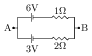# Series and Parallel Arrangements of Cells

## Problems from IIT JEE

Problem (IIT JEE 2011):Two batteries of different emfs and different internal resistances are connected as shown. The voltage across AB (in volts) is,

Solution:Let $I$ be the current in the loop (see figure). The Kirchhoff's loop law, $6-3-2I-1I=0$, gives $I={1}\;\mathrm{A}$. Now, going from B to A along upper branch, we get, $V_B-1+6=V_A$, which gives $V_A-V_B={5}\;\mathrm{V}$.